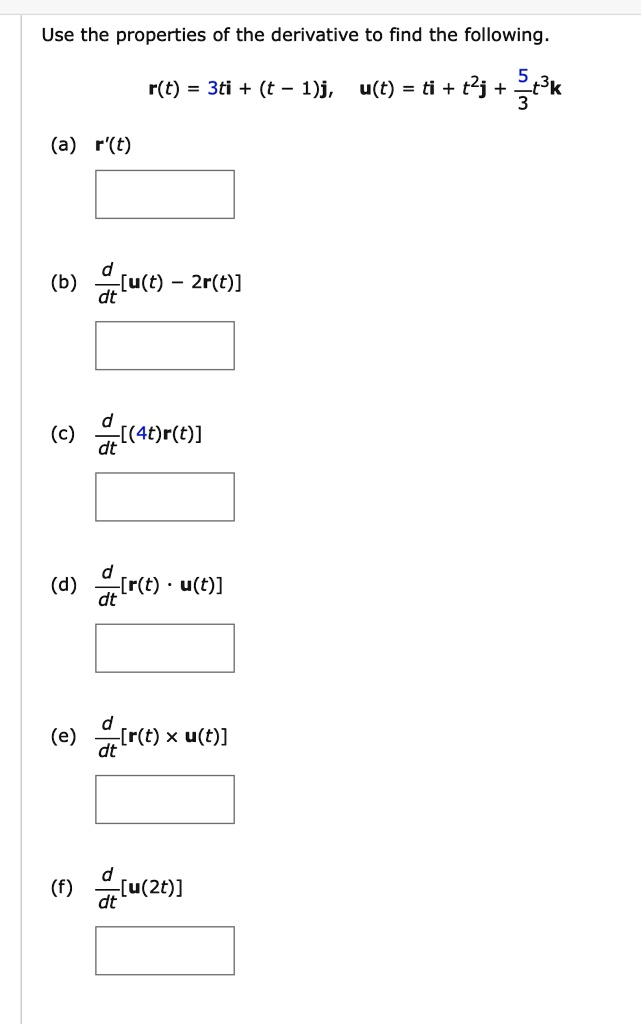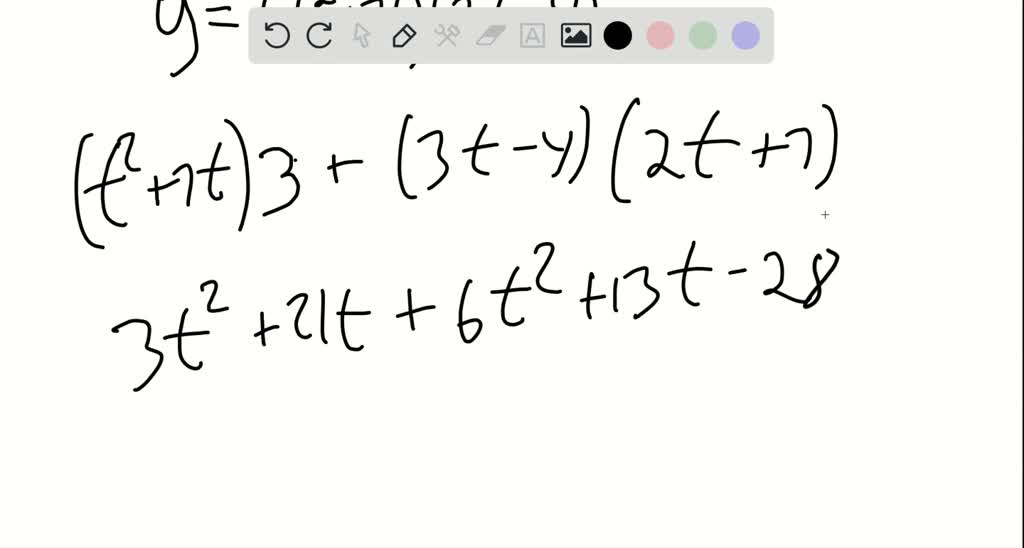5

# Use the properties of the derivative to find the following:r(t) 3ti + (t _ 1)j, u(t) = ti + t2j + Sck (a) r(t)(b) -[u(t) 2r(t)] dt(c) [(4t)r(t)] dt(d)~[r(t) u(t)](e...

## Question

###### Use the properties of the derivative to find the following:r(t) 3ti + (t _ 1)j, u(t) = ti + t2j + Sck (a) r(t)(b) -[u(t) 2r(t)] dt(c) [(4t)r(t)] dt(d)~[r(t) u(t)](e)-[r(t) x u(t)]-[u(2t)]

Use the properties of the derivative to find the following: r(t) 3ti + (t _ 1)j, u(t) = ti + t2j + Sck (a) r(t) (b) -[u(t) 2r(t)] dt (c) [(4t)r(t)] dt (d) ~[r(t) u(t)] (e) -[r(t) x u(t)] -[u(2t)]